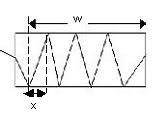# Internal Reflection Problem

• JinM
Attempt at a SolutionMy attempt at the solution was to first find angle of refraction, and I found it using Snell's Law. Theta_r = 30 degreesThen, if you check the figure I attached, I formed an extension and completed the triangle. Using this, I found angle of incidence.Theta_I = 90-30 = 60 degreesAfterwards, I got a triangle of the complete ray. I drew the triangle in the figure I attached. I had Y, which is (l = 3.1 mm). I need to find x, so I used the tangent ratio .x = (3.1 x 10^-3) tan(60f

#### JinM

[SOLVED] Internal Reflection Problem

## Homework Statement

ni = 1
nr = 1.48
theta_I = 50 degrees
l = 3.1 mm
w = 42 cm

Find N (Number of reflections before laser beam finally emerges).

## Homework Equations

Snell's Law: ni sin theta_I = nr sin theta_r

## The Attempt at a Solution

My attempt at the solution was to first find angle of refraction, and I found it using Snell's Law.
Theta_r = 30 degrees

Then, if you check the figure I attached, I formed an extension and completed the triangle. Using this, I found angle of incidence.

Theta_I = 90-30 = 60 degrees

Afterwards, I got a triangle of the complete ray. I drew the triangle in the figure I attached. I had Y, which is (l = 3.1 mm). I need to find x, so I used the tangent ratio .

x = (3.1 x 10^-3) tan(60) = 5.4 x 10^-3 m

Then, I used the following ratio:

N= w/x = (42 x 10^-2) / (5.4 x 10^-3) = 77.8

I rounded the value up to get 78. I don't know, but I'm afraid that I might have done a mistake. Can someone please go over my solution and check if I'm correct?

Thanks,
Jin

EDIT: Whilst someone approves my attachment, here's the problem text:

A laser beam traveling in air strikes the midpoint of one
end of a slab of material as shown in Figure 15-24. The
index of refraction of the slab is 1.48. Determine the
number of internal reflections of the laser beam before
it finally emerges from the opposite end of the slab.

#### Attachments

Last edited:
N= w/x equation; the w is not the width of whole slab. Subtract the distance which first green line(in left picture, upper figure) cross of upper surface to the left of slab.

Hello, and thanks for looking over my post!
I feel foolish that I actually included the width of the slab that contains the refracted ray. The question is asking me for the number of reflections. The first refracted ray wasn't long enough to have the same value of x as the other rays, so I extended it, and the width of the slab. I found the length I needed to subtract from the total width, and then I found the total number of reflections (N). It's about the same answer (77.037). Tell me, am I on the right track?

Also, when I get the value of N, and it's in decimals, I should round up, right?

Thanks again,
Jin

Sorry for double posting, but I need to make sure of my answer. Can someone look over my questions and check if I did it correctly?

Again sorry,
Jin

now i can not check your result but show you a picture below. With right width(w) and rounding the result is ok. Absolutly the units important, all must be the same.I didn't get the chance to thank you for clearing it up: Thanks a lot. :D

Jin